# How To Make A Scatter Plot In Excel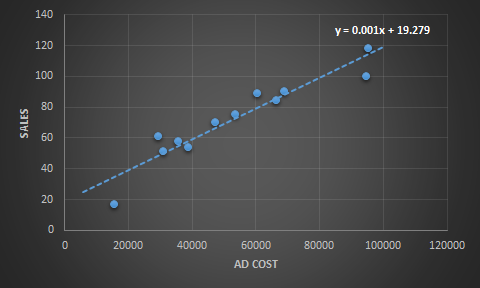What is a Scattered Chart?
A scattered chart is 2D graph representing relation between two or more variables. One variable is shown on X axis and another on Y.

Why Use Scatter Plot?
Use Scatter chart when you want to show relation between two or more variables. The scatter chart can help you learn impact of one variable on other variable. We can visually see the correlation between two or more factors of data gathered. We can add trendlines to estimate future values.

How To Create A Scatter Plot In Excel?
Here, I have prepared data of advertisement cost and sales in different months.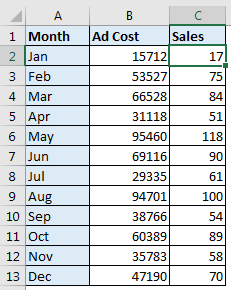We need to see how add cost impacts the sales. To do we can do several analysis tasks but here we will just create scatter plot in excel.

To make scatter plot in excel, follow these steps.

1. Select the two variables data. Here it is Ad Cost and Sales. We will select range B1:C13.
2. Go to insert? Charts? Scattered ? Select ScatterAnd the chart is ready.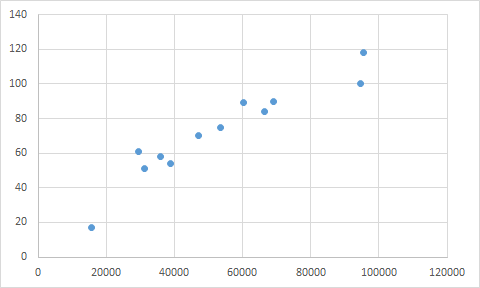Add Axis Titles to Scatter Plot
This chart show sales in relation with advertisement cost. But wait a minute we can’t see axis labels. To add axis labels, follow these steps.

1. Select the scatter plot.
2. Go to Design Tab.
3. Go to Add Chart Element.
4. Click on Axis Titles.
5. Select Primary Horizontal and Primary Vertical, one by one.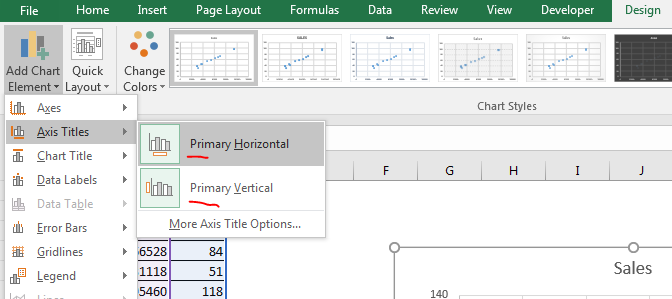1. Now rename them Ad Cost and Sales.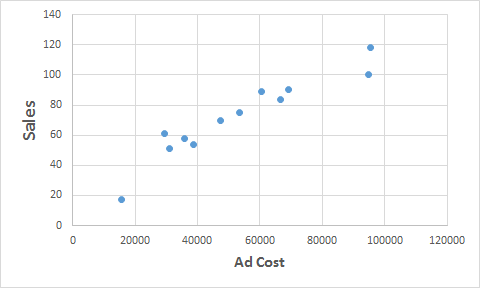Now it is more readable. Now let’s add a trendline to this scatter plot.

1. Select the scatter plot.
2. Go to Design Tab.
3. Click on add chart elements.
4. In the bottom, click on trendline.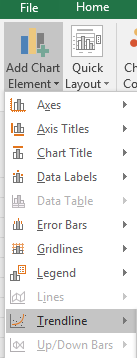1. Click on Linear trendline.
2.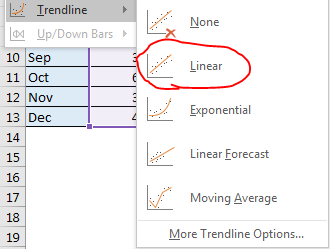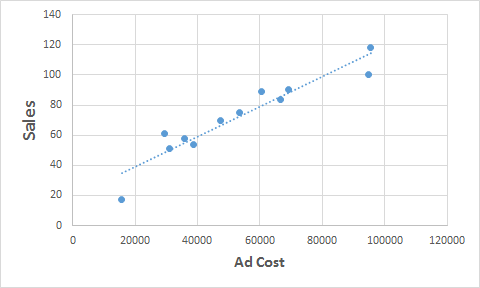Make Scatter Chart Attractive
Now to make this chart little bit readable, format it. Excel provides some preset of designs. I am using one of them here.

1. Go to designs.
2. In charts styles, select the chart style you like.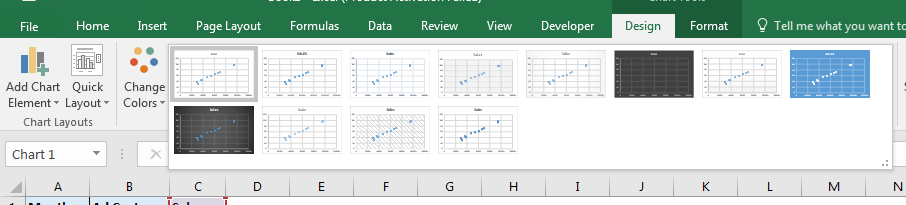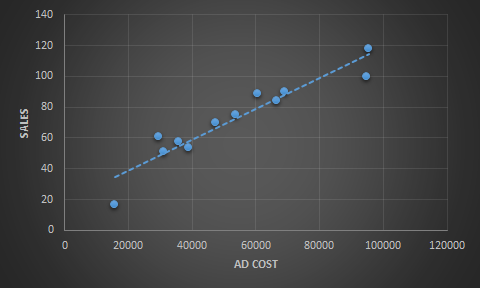Show The xy Equation on Chart
We can also see how sales is related to ad cost in algebraic form. To do so follow these steps:

1. Right click on trendline.
2. Click on Format Trendline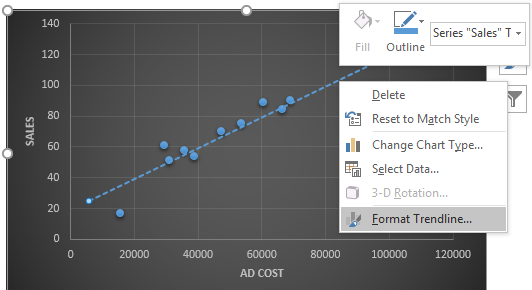1. Click on Trendline Options.
2. In the bottom, check on Display Equation on chart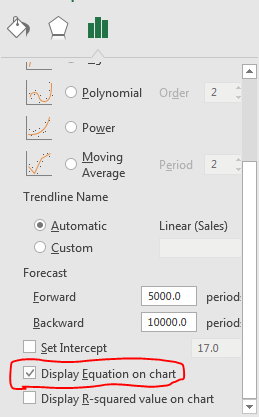Now you will see the regression equation on chart. In this case it Y=0.001x+19.279. This equation tells how much sales is dependent on ad cost. 0.001 is coefficient of x and 19.279 is intercept or slope of the equation.You can change the intercept in scattered chart if you want. Above the Display equation on chart, you can see an option of Set Intercept. Check that to manually set intercept.

So this was a quick tutorial about how to plot scattered chart in excel. Scatter charts has other options too. You can use it to create deviation graphs, to show regression. Many more analytical visualization can be done. In this tutorial we created an xy scatter plot and in excel and learned how to make it more attractive. Let me know if you have any doubts about this tutorial or any other excel related query. The comments section is open for you.

Related Articles:

Regressions in excel 2010

Perform Waterfall Chart in Excel

Perform Pareto Chart and Analysis in Excel

Create Overlay Chart in Excel

Popular Articles :

50 Excel Shortcut to Increase Your Productivity : Get faster at your task. These 50 shortcuts will make you work even faster on Excel.

How to use the VLOOKUP Function in Excel : This is one of the most used and popular functions of excel that is used to lookup value from different ranges and sheets.

How to use the COUNTIF function in Excel : Count values with conditions using this amazing function. You don't need to filter your data to count specific values. Countif function is essential to prepare your dashboard.

How to use the SUMIF Function in Excel : This is another dashboard essential function. This helps you sum up values on specific conditions.

Terms and Conditions of use

The applications/code on this site are distributed as is and without warranties or liability. In no event shall the owner of the copyrights, or the authors of the applications/code be liable for any loss of profit, any problems or any damage resulting from the use or evaluation of the applications/code.